# Calculus 1 : How to find solutions to differential equations

## Example Questions

1 2 7 8 9 10 11 12 13 15 Next →

### Example Question #395 : Equations

Find the derivative of the function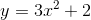.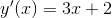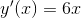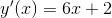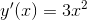Explanation:

To find the derivative of the function, you must use the power rule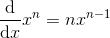.

Using this rule, the correct answer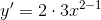is obtained.

### Example Question #1441 : Functions

Find the derivative of the function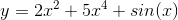.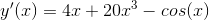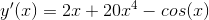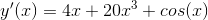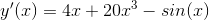Explanation:

To take the derivative of the first two terms, you use the power rule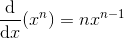.

To take the derivative of sin(x), there is the identity that,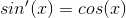.

Using all of the rules and applying,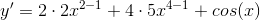.

### Example Question #397 : Equations

Determine the solution to the following: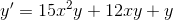with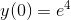.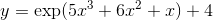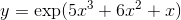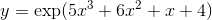The equation is not separable.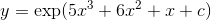Explanation:

Solving a differential equation tends to be quite simple if it is separable. A separable equation is one that can be rewritten in the following form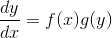.

From here we can manipulate the equation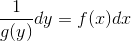and then you can integrate both sides.

We can rewrite the equation as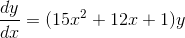and we therefore see that it is separable.

Next we manipulate it to get the the y terms on the left hand side and the x terms on the right hand side like the following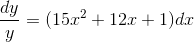.

The next step is to integrate both sides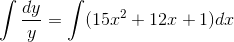.

This gives us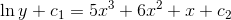,

since the c's are just arbitrary constants we can combine them to get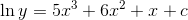.

Since we want to write this as an equation in terms of y we will apply e to both sides.

Therefore we get.

Since the problem gives us an initial condition we solve for c.

Therefore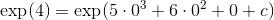so c=4.

Thus the solution is.

### Example Question #398 : Equations

Solve the following differentiable equation: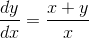with a condition of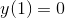.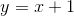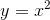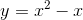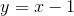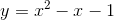Explanation:

When given a first order differential equation to solve, one of the first things to check is that it is homogenous.Homogenous equations are ones in which, given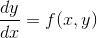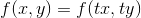given any t.

If an equation is homogenous you can do a substitution of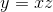and this new equation will be separable.

The first step is to check if the following equation is homogenous,.

Next we show that the equation is homogenous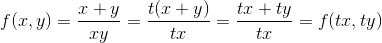.

So we now  replace y with xz. This gives us the following new equation: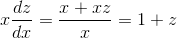.

This is a separable equation which we manipulate and integrate both sides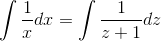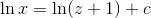.

Thus we get the equation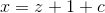,

then plugging y back in we get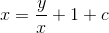.

So our family of equations is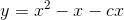yet plugging in the given condition gives us that c=0.

Thus the final solution.

1 2 7 8 9 10 11 12 13 15 Next →

### All Calculus 1 Resources